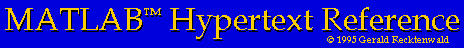# MATLAB Variables

• Overview
• Scalars
• Creating
• Assigning values
• Scalar operations
• Vectors
• Creating
• Assigning values
• Addressing individual elements
• Colon notation
• Vector operations
• Matrices
• creating
• assigning values
• addressing individual elements
• colon notation
• matrix operations
• Strings
• creating
• assigning values
• addressing individual elements
• colon notation
• string operations

## Overview of MATLAB Variables

This section describes the fundamental operations involved in the creation and use of MATLAB variables. Detailed discussions of scalars, vectors, matrices, and strings are provided in separate sections.

MATLAB variables are created when they appear on the left of an equal sign. The generic statement

```	>> variable = expression
```
creates the ``variable'' and assigns to it the value of the expression on the right hand side. You do not need to define or declare a variable before it is used. Matrices do not need to explicitly dimensioned, and MATLAB allows you to increase the size of a matrix as you work. ``Holy FORTRAN, Batman!''

Advanced MATLAB users will point out that the speed of MATLAB functions can be increased by pre-allocating memory for matrices and vectors. This and other performance considerations are discussed elsewhere.

Variable names must begin with an alphanumeric letter. Following that, any number of letters, digits and underscores can be added, but only the first 19 characters are retained. MATLAB variable names are case sensitive so x and X are different variables.

All numerical variables in MATLAB are matrices, a mathematical data type corresponding to a two-dimensional array of numbers. Before performing any calculations with a numeric variable, MATLAB prods and pokes into its contents. MATLAB is very careful to follow the rigorous rules of linear algebra, and it only allows legal operations between matrices, vectors and scalars.

But what about the statement that ``all numerical variables in MATLAB are matrices''?

MATLAB keeps track of the dimension of each variable. Thus, the statement

```	>> x = 2
```
creates a scalar variable x. In MATLAB, a scalar is a variable with one row and one column.

A vector is a matrix with only one row or only one column. The distinction between row and column vectors is crucial. When working with MATLAB you will need to understand how to properly perform linear algebra using scalars, vectors and matrices. MATLAB enforces rules on the use of each of these variables

MATLAB variables may also be strings, which are matrices of individual characters. There is no typographical difference in appearance between numerical variables and string variables. For example, the following statements assign a numerical value to x and a string value to y.

```	>> x = 5.2
>> y = 'Bob'
```
The type of variable (numerical or string) is determined when the variable is created.

### Complex Variables

Numerical matrix elements may be either real or complex. The type of element is initially determined at the time the variable is created. However, matrix elements may change from real to complex if, during an assignment, the right hand side expression evaluates to a complex number. In this sense, matrix elements with real values are simply complex numbers with zero imaginary parts.